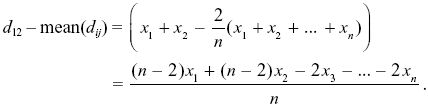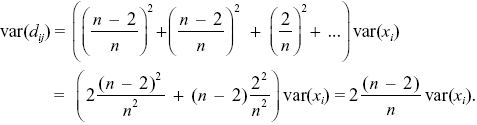NOTE 19U: To see the relation between var(dij) and var(xi), consider the deviation from the mean:The expectation of the square of this deviation is found by summing the expectations of the squares of each term, because the xi are independent of each other (i.e., E[xixj] = 0, E[xi2] = var(xi)). So,